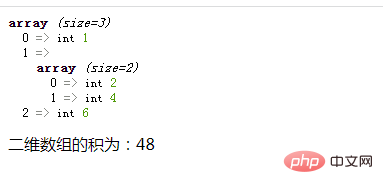# php二维数组怎么求积php入门到就业线上直播课：进入学习

`\$cj=1;`

```foreach(\$arr as \$v){
//循环体代码
}```

• 如果不是数组类型，则利用“*=”运算符将不是的外层元素相乘求积

• 如果是数组类型，则使用array_product()函数计算该内层数组中所有元素的积，并与外层元素和相乘在一起即可。

```if(is_array(\$v)){
\$cj*=array_product(\$v);
}else{
\$cj*=\$v;
}```

```<?php
\$arr = array(1,array(2,4),6);
var_dump(\$arr);
\$cj=1;
foreach(\$arr as \$v){
if(is_array(\$v)){
\$cj*=array_product(\$v);
}else{
\$cj*=\$v;
}
}
echo "二维数组的积为：".\$cj;
?>```1/1